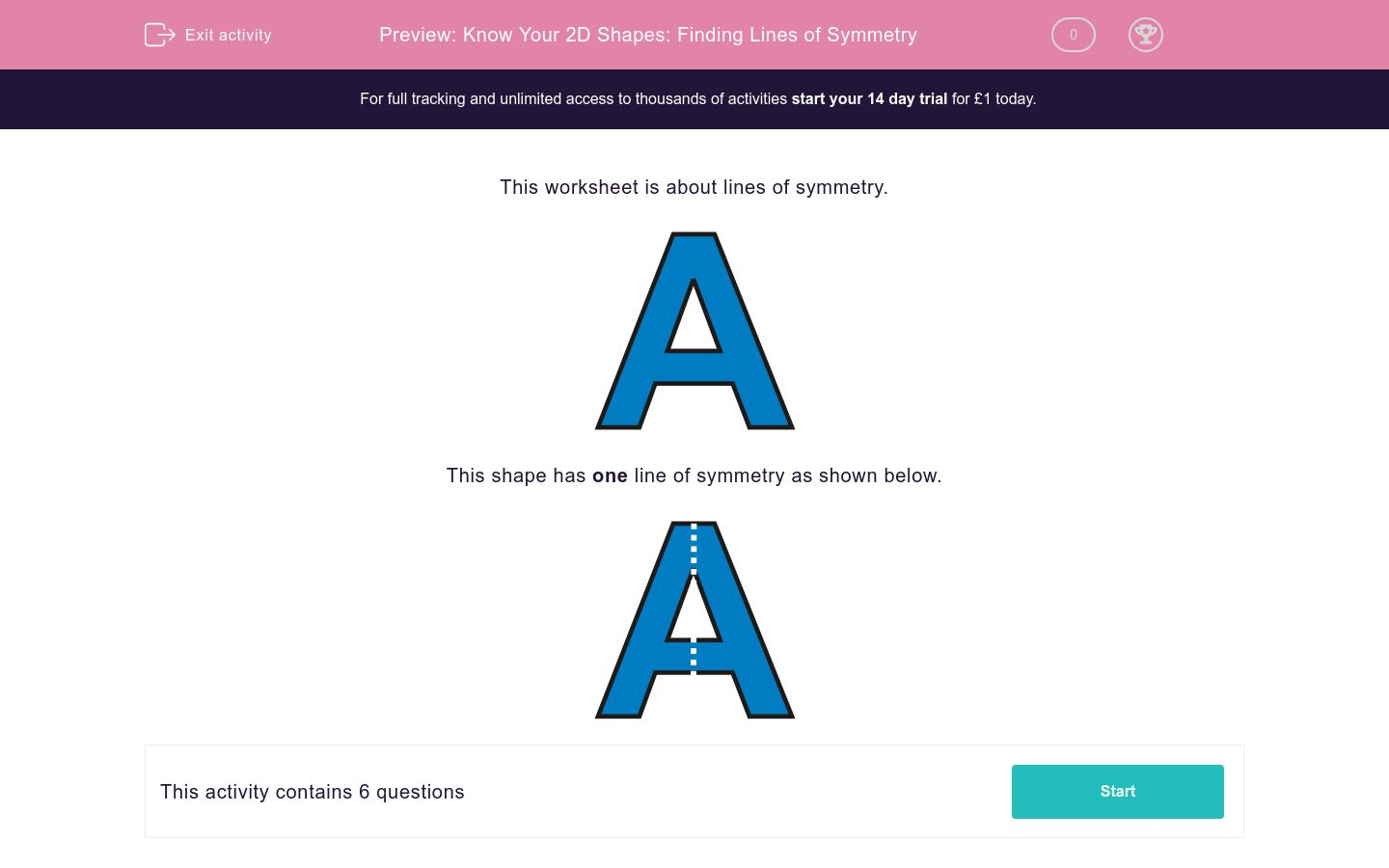# Know Your 2D Shapes: Finding Lines of Symmetry

In this worksheet, students state how many lines of symmetry a shape has.Key stage:  KS 2

Curriculum topic:   Maths and Numerical Reasoning

Curriculum subtopic:   Symmetry

Difficulty level:### QUESTION 1 of 10

This worksheet is about lines of symmetry.This shape has one line of symmetry as shown below.How many lines of symmetry can be drawn on this shape?1

2

3

How many lines of symmetry can be drawn on this shape?1

2

3

How many lines of symmetry can be drawn on this shape?1

2

3

How many lines of symmetry can be drawn on this shape?1

2

3

How many lines of symmetry can be drawn on this shape?3

4

5

How many lines of symmetry can be drawn on this shape?1

2

3

• Question 1

How many lines of symmetry can be drawn on this shape?2
EDDIE SAYS• Question 2

How many lines of symmetry can be drawn on this shape?1
EDDIE SAYS• Question 3

How many lines of symmetry can be drawn on this shape?1
EDDIE SAYS• Question 4

How many lines of symmetry can be drawn on this shape?1
EDDIE SAYS• Question 5

How many lines of symmetry can be drawn on this shape?5
EDDIE SAYS• Question 6

How many lines of symmetry can be drawn on this shape?2
EDDIE SAYS---- OR ----